# Civil Engineering - UPSC Civil Service Exam Questions

16.

A gradually applied load W is suspended by with ropes AB and CD as shown in the figure. The wires AB and CD, made of the same material and of the same cross-section are connected to a rigid block from which the load W is suspended in such a way that both the ropes stretch by the same amount. If the stress in AB and CD are p1 and p2 respectively, then the ratio - will be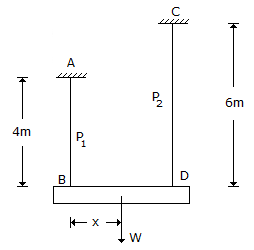A.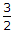B.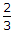C.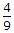D.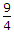Explanation:

No answer description available for this question. Let us discuss.

17.

For a state of plane stress σ1 = σx = 40 MPa, and σ2 = σy = 20MPa. What are the values of the maximum in-plane shearing stress and absolute maximum shearing stress ?

 A. (± 10, 20) MPa B. (± 10, 10) MPa C. (± 20, 10) MPa D. (± 20, 20) MPa

Explanation:

No answer description available for this question. Let us discuss.

18.

A T-beam roof section has the following particulars :
Thickness of slab 100 mm
Width of rib 300 mm
Depth of beam 500 mm
Centre to centre distance of beams 3.0 m
Effective span of beams 6.0 m
Distance between points of contraflexure is 3.60 m.
The effective width of flange of the beam is

 A. 3000 mm B. 1900 mm C. 1600 mm D. 1500 mm

Explanation:

No answer description available for this question. Let us discuss.

19.

A dry sand specimen is put through a triaxial test. The cell pressure is 50 kPa and the deviator stress at failure is 100 Pa. The angle of internal friction for the sand specimen is

 A. 15° B. 30° C. 37° D. 45°

Explanation:

No answer description available for this question. Let us discuss.

20.

A channel of bed slope 0.0009 carries a discharge of 30 m3/s when the depth of flow is 1.0 m. What is the discharge carried by an exactly similar channel at the same depth of flow if the slope is decreased to 0.0001 ?

 A. 10 m3/s B. 15 m3/s C. 60 m3/s D. 90 m3/s